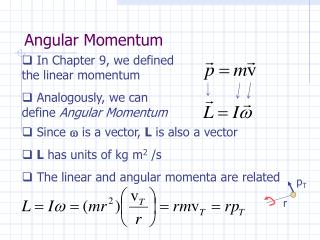DownloadDownload PresentationAngular Momentum

# Angular Momentum

Télécharger la présentation## Angular Momentum

- - - - - - - - - - - - - - - - - - - - - - - - - - - E N D - - - - - - - - - - - - - - - - - - - - - - - - - - -
##### Presentation Transcript

1. Angular Momentum • In Chapter 9, we defined the linear momentum • Analogously, we can define Angular Momentum • Since  is a vector, L is also a vector • L has units of kg m2 /s • The linear and angular momenta are related pT r

2. L gives us another way to express the rotational motion of an object • For linear motion, if an external force was applied for some short time duration, a change in linear momentum resulted • Similarly, if an external torque is applied to a rigid body for a short time duration, its angular momentum will change • If • This is the Principle of Conservation of Angular Momentum

3. How to interpret this? Say the moment of inertia of an object can decrease. Then, its angular speed must increase. (Example 11-10) Example Problem For a certain satellite with an apogee distance of rA=1.30x107 m, the ratio of the orbital speed at perigee to the orbital speed at apogee is 1.20. Find the perigee distance rP.  Not uniform circular motion

4. Satellites generally move in elliptical orbits. Also, the tangential velocity is not constant. • If the satellite is ``circling’’ the vP   A P vA rA rP Earth, the furthest point in its orbit from the Earth is called the ``apogee.’’ The closest point the ``perigee.’’ For the Earth circling the sun, the two points are called the ``aphelion’’ and ``perihelion.’’

5. Given: rA = 1.30x107 m, vP/vA = 1.20. Find: rP ? Method: Apply Conservation of Angular Momentum. The gravitational force due to the Earth keeps the satellite in orbit, but that force as a line of action through the center of the orbit, which is the rotation axis of the satellite. Therefore, the satellite experiences no external torques.

6. Summary TranslationalRotational x displacement  v velocity  a acceleration  F cause of motion  m inertia I F=ma 2nd Law  =I  Fs work   1/2mv2 KE 1/2I 2 p=mv momentum L=I 